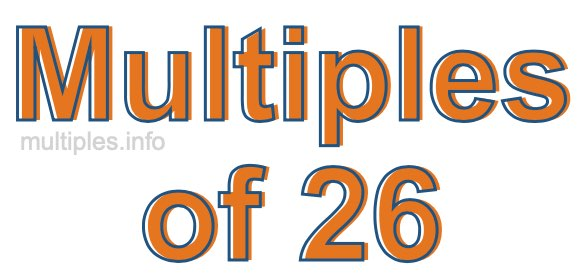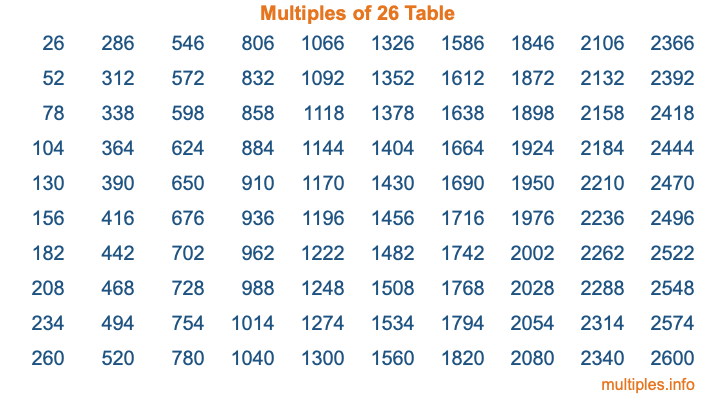Multiples of 26Welcome to the Multiples of 26 page. Here we will first teach you everything you will ever need to know about the multiples of 26, and then give you a study guide summary of everything we taught you to make sure you remember it all. Use this page to look up facts and learn information about the multiples of 26. This page will make you a multiples of twenty-six expert!

Definition of Multiples of 26
Multiples of 26 are all the numbers that when divided by 26 equal an integer. Each of the multiples of 26 are called a multiple. A multiple of 26 is created by multiplying 26 by an integer.

Therefore, to create a list of multiples of 26, you start with 1 multiplied by 26, then 2 multiplied by 26, then 3 multiplied by 26, and so on for as long as you want. Thus, the list of the first five multiples of 26 is 26, 52, 78, 104, and 130. To see a larger list of multiples of 26, see the printable image of Multiples of 26 further down on this page. We also have a category where you can choose any nth multiple of 26.

Multiples of 26 Checker
The Multiples of 26 Checker below checks to see if any number of your choice is a multiple of 26. In other words, it checks to see if there is any number (integer) that when multiplied by 26 will equal your number. To do that, we divide your number by 26. If the the quotient is an integer, then your number is a multiple of 26.

Is  a multiple of 26?

Least Common Multiple of 26 and ...
A Least Common Multiple (LCM) is the lowest multiple that two or more numbers have in common. This is also called the smallest common multiple or lowest common multiple and is useful to know when you are adding our subtracting fractions. Enter one or more numbers below (26 is already entered) to find the LCM.

Check out our LCM Calculator if you need more details about the Least Common Multiple or if you need the LCM for different numbers for adding and subtraction fractions.

nth Multiple of 26
As we stated above, 26 is the first multiple of 26, 52 is the second multiple of 26, 78 is the third multiple of 26, and so on. Enter a number below to find the nth multiple of 26.

th multiple of 26

Multiples of 26 vs Factors of 26
26 is a multiple of 26 and a factor of 26, but that is where the similarities end. All postive multiples of 26 are 26 or greater than 26. All positive factors of 26 are 26 or less than 26.

Below is the beginning list of multiples of 26 and the factors of 26 so you can compare:

Multiples of 26: 26, 52, 78, 104, 130, etc.

Factors of 26: 1, 2, 13, 26

As you can see, the multiples of 26 are all the numbers that you can divide by 26 to get a whole number. The factors of 26, on the other hand, are all the whole numbers that you can multiply by another whole number to get 26.

It's also interesting to note that if a number (x) is a factor of 26, then 26 will also be a multiple of that number (x).

Multiples of 26 vs Divisors of 26
The divisors of 26 are all the integers that 26 can be divided by evenly. Below is a list of the divisors of 26.

Divisors of 26: 1, 2, 13, 26

The interesting thing to note here is that if you take any multiple of 26 and divide it by a divisor of 26, you will see that the quotient is an integer.

Multiples of 26 Table
Below is an image of the first 100 multiples of 26 in a table. The table is in chronological order, column by column. The first column has the first ten multiples of 26, the second column has the next ten multiples of 26, and so on.The Multiples of 26 Table is also referred to as the 26 Times Table or Times Table of 26. You are welcome to print out our table for your studies.

Negative Multiples of 26
Although not often discussed or needed in math, it is worth mentioning that you can make a list of negative multiples of 26 by multiplying 26 by -1, then by -2, then by -3, and so on, to get the following list of negative multiples of 26:

-26, -52, -78, -104, -130, etc.

Multiples of 26 Summary
Below is a summary of important Multiples of 26 facts that we have discussed on this page. To retain the knowledge on this page, we recommend that you read through the summary and explain to yourself or a study partner why they hold true.

There are an infinite number of multiples of 26.

A multiple of 26 divided by 26 will equal a whole number.

26 divided by a factor of 26 equals a divisor of 26.

The nth multiple of 26 is n times 26.

The largest factor of 26 is equal to the first positive multiple of 26.

26 is a multiple of every factor of 26.

26 is a multiple of 26.

A multiple of 26 divided by a divisor of 26 equals an integer.

26 divided by a divisor of 26 equals a factor of 26.

Any integer times 26 will equal a multiple of 26.

Multiples of a Number
Here you can get the multiples of another number, all with the same attention to detail as we did for multiples of 26 on this page.

Multiples of
Multiples of 27
Did you find our page about multiples of twenty-six educational? Do you want more knowledge? Check out the multiples of the next number on our list!